# NCERT Solutions for Class 7 Maths Chapter 8 Comparing Quantities Ex 8.2

NCERT Solutions for Class 7 Maths Chapter 8 Comparing Quantities Ex 8.2

### NCERT Solutions for Class 7 Maths Chapter 8 Comparing Quantities Ex 8.2

NCERT Solutions for Class 7 Maths Chapter 8 Comparing Quantities Exercise 8.2

Ex 8.2 Class 7 Maths Question 1.
Convert the given fractional numbers to per cents:Solution: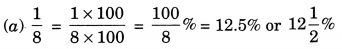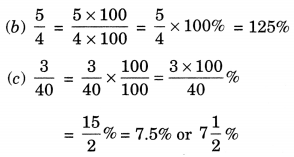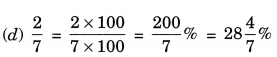Ex 8.2 Class 7 Maths Question 2.
Convert the given decimal fractions to per cents:
(а) 0.65
(b) 2.1
(c) 0.02
(d) 12.35
Solution:Ex 8.2 Class 7 Maths Question 3.
Estimate what part of the figures is coloured and hence find the per cent which is coloured.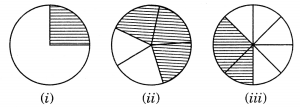Solution:
(i) Fraction of coloured part = 1/4
∴ Percentage of coloured parts 100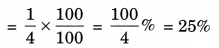(ii) Fraction of coloured part = 3/6
∴ Percentage of coloured parts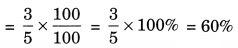(iii) Fraction of coloured part = 6/8
∴ Percentage of coloured parts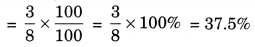Ex 8.2 Class 7 Maths Question 4.
Find:
(a) 15% of 250
(b) 1% of 1 hour
(c) 20% of ₹ 2500
(d) 75% of 1 kg
Solution:
(a) 15% of 250 = 15/100×250=75/2=37.5
(b) 1% of 1 hour = 1% of 60 minutes [∵ 1 h = 60 min.]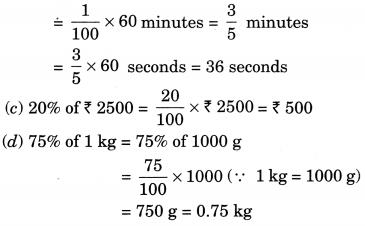Ex 8.2 Class 7 Maths Question 5.
Find the whole quantity if
(a) 5% of it is 600
(b) 12% of it is? 1080
(c) 40% of it is 500 km
(d) 70% of it is 14 minutes
(e) 8% of it is 40 litres
Solution:
Let the required whole quantity be x.
(a) 5% of x = 600Thus the required whole quantity is 12,000.

(b) 12% of x = ₹ 1080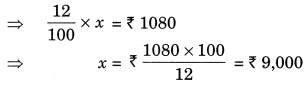Thus, the required quantity is ₹ 9,000.

(c) 40% of x = 500 km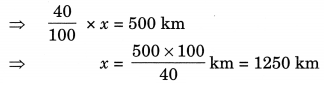Thus, the required quantity = 1250 km.

(d) 70% of x = 14 minutes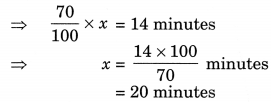Thus, the required quantity = 20 minutes,

(e) 8% of x = 40 litre 8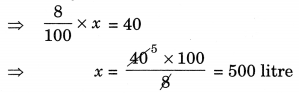Thus, the required quantity = 500 litres

Ex 8.2 Class 7 Maths Question 6.
Convert given per cents to decimal fractions and also to fractions in simplest forms:
(a) 25%
(b) 150%
(c) 20%
(d) 5%
Solution: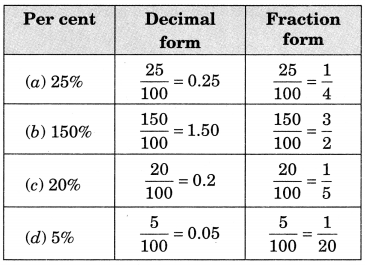Ex 8.2 Class 7 Maths Question 7.
In a city, 30% are females, 40% are males and remaining are children. What per cent are children?
Solution:
Given: 30% are females
40% are males
Total Percentage of females and males
= 30% + 40% = 70%
∴ Percentage of children
= (100 – 70)% = 30%

Ex 8.2 Class 7 Maths Question 8.
Out of 15,000 voters in a constituency, 60% voted. Find the Percentage of voters who did not vote. Can you now find how many actually did not vote?
Solution:
Total number of voters = 15,000
Percentage of the voters who voted = 60%
∴ Percentage of the voters who did not vote
= (100 – 60)% = 40%
Actual number of voters who did not vote
= 40% of 15,000
=40/100×15,000=6,000

Ex 8.2 Class 7 Maths Question 9.
Meena saves ₹ 400 from her salary. If this is 10% of her salary. What is her salary?
Solution:
Let Meena’s salary by ₹ x.
∴ 10% of x = ₹ 400Thus, her salary is ₹ 4000.

Ex 8.2 Class 7 Maths Question 10.
A local cricket team played 20 matches in one season. It won 25% of them. How many matches did they win?
Solution:
Number of matches played by the cricket team = 20
Percentage of the matches won by them = 25%
i.e. 25/100×20=5 matches
Thus, the number of matches won by them = 5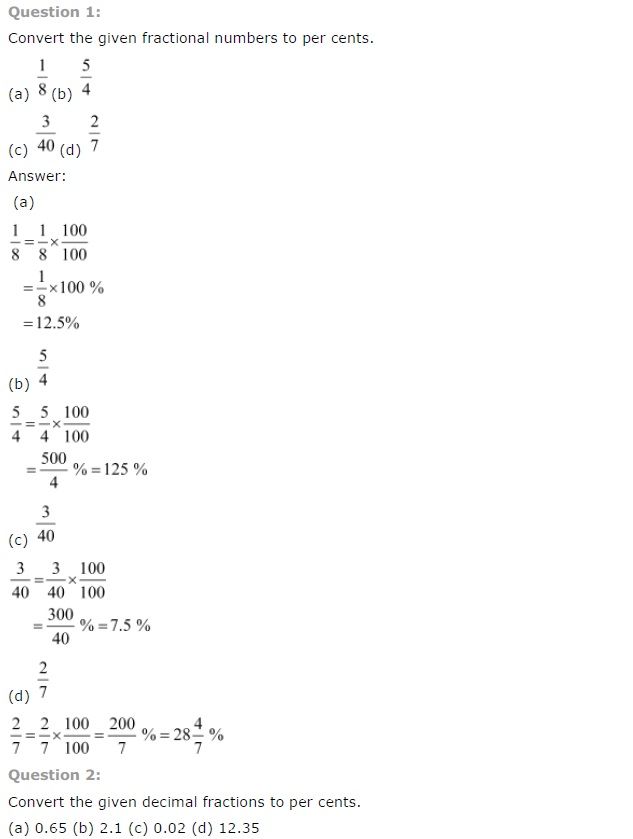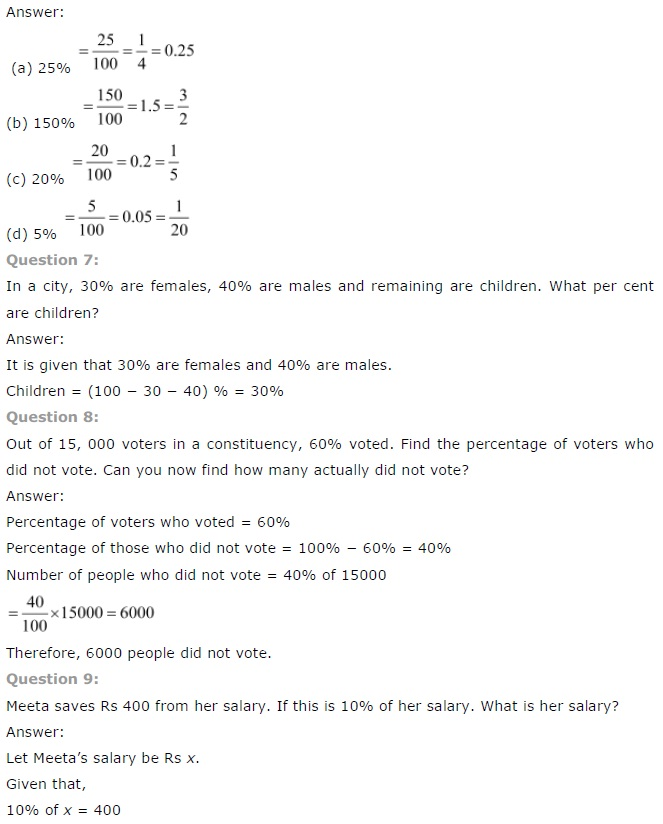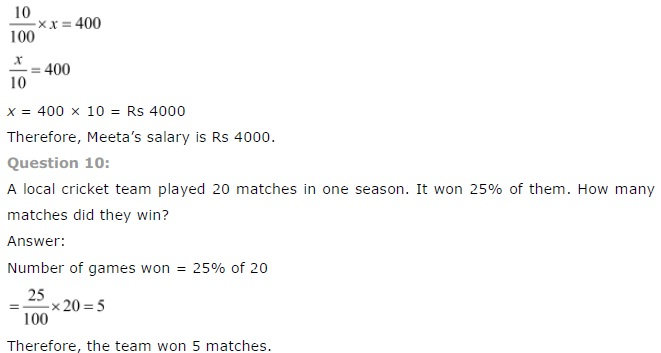## SabDekho

The Complete Educational Website